Computing the Definite Integral of a Polynomial

We want to focus on the definite integral of a polynomial function. These arise very commonly in calculus, so here are detailed solutions to two problems, one multiple-choice and one free-response, involving a definite integral of polynomial.

Free-Response Definite Integrals:

You will not commonly be asked to evaluate common definite integrals on the free-response, but rather you will be asked to find an area or compute a volume, which will require computing a common definite integral. Suppose we want to compute the volume of the solid obtained by revolving the function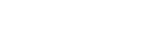about the x-axis:The cross sections when cutting perpendicular to the x-axis are circles with radius given by the function. The definite integral that needs to be evaluated is,  since this is the area of a circle multiplied by the length of the interval from -6 to 6. We compute:Therefore to compute the integral we compute the sum of the integrals of the individual terms, since polynomials are sums of continuous functions:Recall the Fundamental Theorem of Calculus (FTC):

THEOREM: If v(x) is a continuous function with an antiderivative V(x), then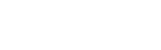where ,  are in the domain of v(x).

The FTC says that we can pick any old antiderivative V(x) for v(x), so we need to compute a string of antiderivatives for the integrands of the terms in the sum. In the previous post we discussed but did not state:

The Power Rule: The derivative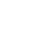‘=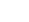We used this to find that the integral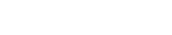, and since we only need one antiderivative to evaluate definite integrals, we can take  for use in this case.

Therefore we can evaluate (using the fact that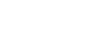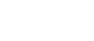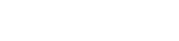and the FTC):You can use your calculator to get 723.823 units cubed.

Multiple-Choice Definite Integrals:

Here is a sample of a typical multiple-choice question asking for you to formulate a definite integral based on the same concept discussed above.

Question: A solid is generated by revolving the region enclosed by the function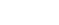, and the lines x=2, x=3, y=1 about the x-axis. Which of the following definite integrals gives the volume of the solid? (Hint: Draw a picture)The idea for this problem is to recognize that this solid is a difference of integrals. Suppose that we had the volume of the functionwhen bounded by the lines  x = 2, x = 3, and rotated about the x-axis—then we would have the volume of the following solid:Given this volume, we would only need to subtract the volume of the following figure, derived by rotating y=1 bounded by x=2, x=3, about the x-axis:From the upper volume, with radius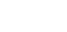:Therefore we need to subtract the two integrals, however using the integral laws we can express this in the form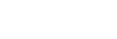, which we follow up by substitution of our names for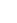,:This has the value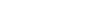.

Improve your SAT or ACT score, guaranteed. Start your 1 Week Free Trial of Magoosh SAT Prep or your 1 Week Free Trial of Magoosh ACT Prep today!Author

•In addition to blogging about AP Calculus, Oakland resident Chris Wirick has played the oboe in Beijing and Berlin, studied math and cognitive science (the other CS) at Cal Berkeley, and can’t stop gardening, cooking and eating new foods.